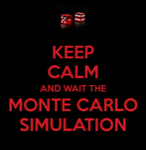Cococubed.com Monte Carlo White Dwarfs

Home

Astronomy research
Software Infrastructure:
MESA
FLASH
STARLIB
MESA-Web
starkiller-astro
My instruments
White dwarf supernova:
Remnant metallicities
Colliding white dwarfs
Merging white dwarfs
Ignition conditions
Metallicity effects
Central density effects
Detonation density effects
Tracer particle burning
Subsonic burning fronts
Supersonic burning fronts
W7 profiles
Massive star supernova:
Rotating progenitors
3D evolution
26Al & 60Fe
44Ti, 60Co & 56Ni
Effects of 12C +12C
SN 1987A light curve
Constraints on Ni/Fe ratios
An r-process
Neutron Stars and Black Holes:
Black Hole Mass Gap
Compact object IMF
Stars:
Neutrino HR diagram
Pulsating white dwarfs
Pop III with JWST
Monte Carlo massive stars
Neutrinos from pre-SN
Pre-SN variations
Monte Carlo white dwarfs
SAGB stars
Classical novae
He shell convection
Presolar grains
He burn on neutron stars
BBFH at 40 years
Chemical Evolution:
Iron Pseudocarbynes
Hypatia catalog
Zone models H to Zn
Mixing ejecta
γ-rays within 100 Mpc
Thermodynamics & Networks
Stellar EOS
12C(α,γ)16O Rate
Proton-rich NSE
Reaction networks
Bayesian reaction rates
Verification Problems:
Validating an astro code
Su-Olson
Cog8
RMTV
Sedov
Noh
Software instruments
Presentations
Illustrations
Public Outreach
Education materials
2022 ASU Solar Systems Astronomy
2022 ASU Energy in Everyday Life

AAS Journals
2022 Earendel, A Highly Magnified Star
2022 TV Columbae, Micronova
2022 White Dwarfs and 12C(α,γ)16O
2022 MESA in Don't Look Up
2022 MESA Marketplace
2022 MESA Summer School
2022 MESA Classroom
2021 Bill Paxton, Tinsley Prize

Contact: F.X.Timmes
my one page vitae,
full vitae,
research statement, and
teaching statement.
Properties Of Carbon-Oxygen White Dwarfs From Monte Carlo Stellar Models (2016)

In this article we investigate properties of carbon-oxygen white dwarfs with respect to the composite uncertainties in the reaction rates using the stellar evolution toolkit, Modules for Experiments in Stellar Astrophysics (MESA) and the probability density functions in the reaction rate library STARLIB. These are the first Monte Carlo stellar evolution studies that use complete stellar models.

Focusing on 3 M$_{\odot}$ models evolved from the pre main-sequence to the first thermal pulse, we survey the remnant core mass, composition, and structure properties as a function of 26 STARLIB reaction rates covering hydrogen and helium burning using a Principal Component Analysis and Spearman Rank-Order Correlation. Relative to the arithmetic mean value, we find the width of the 95% confidence interval to be $\Delta {\rm M}_{{\rm 1TP}} \simeq 0.019 \, {\rm M}_{\odot}$ for the core mass at the first thermal pulse, $\Delta t_{{\rm 1TP}} \simeq$ 12.50 Myr for the age, $\Delta \log(T_c/K) \simeq$ 0.013 for the central temperature, $\Delta \log(\rho_c / {\rm g \ cm^{-3}}) \simeq$ 0.060 for the central density, $\Delta Y_{{\rm e,c}} \simeq$ 2.6×10-5 for the central electron fraction, $\Delta X_c (^{22}{\rm Ne}) \simeq$ 5.8×10-4, $\Delta X_c(^{12}{\rm C}) \simeq$ 0.392, and $\Delta X_c(^{16}{\rm O}) \simeq$ 0.392. Uncertainties in the experimental $^{12}{\rm C}(\alpha,\gamma)^{16}{\rm O}$, triple-$\alpha$, and $^{14}{\rm N}(p,\gamma)^{15}{\rm O}$ reaction rates dominate these variations.

We also consider a grid of 1 to 6 $M_{\odot}$ models evolved from the pre main-sequence to the final white dwarf to probe the sensitivity of the initial-final mass relation to experimental uncertainties in the hydrogen and helium reaction rates.